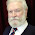Wednesday, June 22, 2016

THE ILLUSION OF SPACE-TIME

TIME, CONSCIOUSNESS
AND REALITY
WHERE TO DRAW THE LINE BETWEEN THE REAL AND THE UNREAL
THE DIMENSIONALITY OF TIME
EINSTEIN’S RELATIVITY RECOGNIZES TIME AS THE FOURTH DIMENSION IN THE 4-D DOMAIN OF SPACE-TIME. AS SUCH, TIME IS IRREVOCABLY BOUND TO THE THREE DIMENSIONS OF SPACE MATHEMATICALLY, SUCH THAT THE SPEED OF LIGHT IS ALWAYS THE SAME REGARDLESS OF THE MOTION OF THE OBSERVER RELATIVE TO THE LOCATION OF ANY GIVEN SPACE-TIME EVENT.
THE MOST IMPORTANT RESULT OF THIS INVARIANT RELATIONSHIP IS THE FACT THAT SPACE-TIME, AND CONSEQUENTLY SPACE AND TIME HAVE NO EXISTENCE APART FROM MASS AND ENERGY. THUS EMPTY SPACE WITHOUT AN OBJECT, AND TIME WITHOUT AN EVENT, HAVE NO PLACE IN REALITY.
THIS RAISES AN IMPORTANT QUESTION: WHAT IS REAL AND WHAT IS NOT?

TIME IS AN ILLUSION – A. EINSTEIN
TO UNDERSTAND WHY TIME, AS WE THINK OF IT, IS AN ILLUSION WE MUST CONSIDER TIME AS WHAT IT IS MATHEMATICALLY: IT IS THE FOURTH DIMENSION
DIMENSIONAL DOMAINS HAVE A NUMBER OF INVARIANT FEATURES PROVED BY THE CALCULUS OF DISTINCTIONS. ONE OF THEM WAS MENTIONED IN THE FOURTH SLIDE OF MY THIRD PRSENTATION.
TO UNDERSTAND WHY TIME, AS WE THINK OF IT, IS AN ILLUSION WE MUST CONSIDER TIME AS WHAT IT IS MATHEMATICALLY: IT IS THE FOURTH DIMENSION
DIMENSIONAL DOMAINS HAVE A NUMBER OF INVARIANT FEATURES PROVED BY THE CALCULUS OF DISTINCTIONS.

WHAT ARE DIMENSIONS?
Dimensions are distinctions that are measureable in variables of extent.  All other types of distinctions are not dimensional. This gives us a mathematically precise definition of the term dimension.
An n-dimensional domain is a domain of with exactly n dimensions.
Every n-dimensional domain is contained within an n+1-dimensional domain.

E=mc2
and
the Second Dimension of Time
EINSTEIN’S FAMOUS EQUATION IS MUCH MORE THAN JUST AN EXPRESSION OF THE MATHEMATICAL CONVERSION OF MATTER TO ENERGY
E=mc2 ALSO EXPRESSES A DIMENSIONAL RELATIONSHIP BETWEEN MASS, ENERGY, SPACE AND TIME
THE SPEED OF LIGHT, SYMBOLIZED BY LOWERCASE ‘c’, IS DEFINED AS THE DISTANCE TRAVELLED BY A PHOTON OF LIGHT DIVIDED BY THE LENGTH OF TIME IT TAKES IT TO TRAVEL THAT DISTANCE. MATHEMATICALLY: c = Δx/Δt
c = Δx/Δt → c2 = (Δx)2/(Δt)2
KEEP IN MIND THAT A IN 3-D DOMAIN, DISTANCE SQUARED IS AN AREA. SIMILARILY, IN A 5-D DOMAIN, LINEAR TIME SQUARED IS AN AREA OF TIME
ANOTHER DIMENSIONAL DOMAIN INVARIANCE PROVED BY THE CALCULUS OF DISTINCTIONS, IS THE FACT THAT, IN A QUANTIZED REALITY, A DISTINCTION IS ONLY ABLE TO HAVE  CONTENT IF IT HAS THREE OR MORE DIMENSIONS
THEREFORE, AN EVENT OCCURRING IN TIME MUST OCCUPY THREE DIMENSIONS OF TIME TO BE A REAL EVENT
FINALLY, THE EXISTENCE OF A THIRD DIMENSION OF TIME FULFILLS THE REQUIREMENT THAT EVERY N-DIMENSIONAL DOMAIN IS CONTAINED WITHIN AN N+1-DIMENSIONAL DOMAIN.

CONCLUSIONS:
THE REAL AND THE UNREAL
TIME AND SPACE ARE UNREAL IN SO MUCH AS THEY HAVE NO EXISTENCE APART FROM EXTENDED MASS, ENERGY AND CONSCIOUSNESS. THEIR OBJECTIVITY IS AN ILLUSION CREATED BY THE INTERACTION OF THE REAL SUBSTANCES EXISTING IN THE STRUCTURES OF 9-D FINITE REALITY

CONSCIOUS, ON THE OTHER HAND, IS REAL BECAUSE, AS PRIMARY ONSCIOUSNESS, IT CONTAINS THE 3-D STRUCTURES OF MASS, ENERGY AND EVENTS, AND IT IS NECESSARY FOR ANY MEANINGFUL ATOMIC STRUCCCTURE TO FORM, AND AS INDIVIDUALIZED CONSCIOUSNESS (US), IT CONTAINS FINITE 3-D TRUE UNITS OF THE THIRD FORM OF REALITY (GIMMEL).

GIMMEL IS NEITHER MASS NOR ENERGY, AND IT CONVEYS THE CHARACTERISTICS OF CONSCIOUSNESS TO THE STRUCTURE OF SENTIENT LIFE FORMS.

1.2.3.